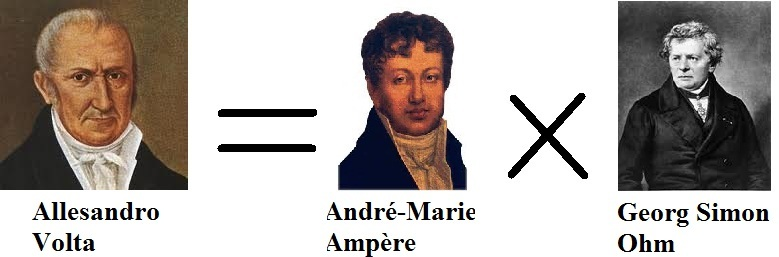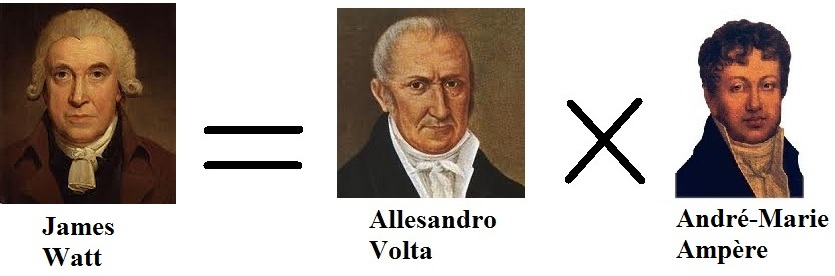﻿Definition of electrical resistance

# The didactics of Ohm's LawPhysically seen: each curve is a straight line,
if you look at it detailed enough.

Ohm's law
potential difference or voltage = strength of current × resistance was called Ohm's Law always.
However this formula is not a law; not at all!
In fact voltage = strength of current × resistance is nothing but a definition;
the definition of resistance.

Voltage = strength of current × resistance would be a law
if and only if the resistance stayed constant whatever the conditions of the resistor.
However nature not dishes out ever this requirement.
The ideal resistance does not exist so neither does Ohm's Law.
Unfortunately voltage and strength of current are not proportional!
The resistance of a resistor does not exist.

Many teachers have been doing their best to try
to let sign by their students
the graph showing the relationship between voltage and current strength
as a straight line. I confess: I did it too.
Nature is here substantially violated and didactics is therefore misleading.

The definition of resistance
From a didactical perspective I suggest
to avoid the expression Ohm's law and
to talk about the definition of resistance.